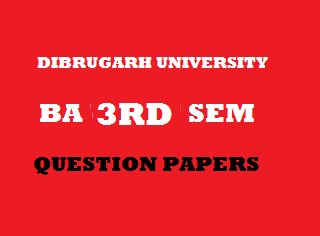## Thursday, January 03, 20192014
(November)
EDUCATION
(General)
Course: 301
(Measurement and Evaluation in Education)
Full Marks: 80
Pass Marks: 32
Time: 3 hours
The figures in the margin indicate full marks for the questions

1. Give short answers/Selected and write the correct answer to the following questions: 1x8=8
1. Who founded the psychological laboratory for the first time?
2. The degree to which a test measures what it purports to measure is called
1. Reliability of the test.
2. Objectivity of the test.
3. Validity of the test.
4. Norms of the test.
1. Write the full form of CCE.
2. Who introduced first the concept of Intelligence Quotient?
3. Who developed the Thematic Apperception Test (TAT)?
4. Mention one property of arithmetic mean.
5. Calculate the range of the following set of scores:
17, 09, 22, 16, 19, 20, 13, 23
1. What is meant by negative correlation?
2. What are meant by measurement and evaluation of education? Describe their relationship with appropriate examples. 4+4+6=14
3. What is meant by norms of a test? Describe different types of norms of a test. 2+8=10
4. Describe the Stanford Revision of Binet-Simon scale of 1916. 9
Or
What is rating scale? Describe the various types of rating scale. 3+6=9
5. What is meant by statistics? Explain its need and importance in the field of education and psychology.     2+5+5=12
6. Write short notes on: 5x3=15
1. Skewness.
2. Thematic Apperception Test.
3. Validity of a Test.

7. Plot a histogram from the following distribution table: 4
 Class interval Frequency 90-9485-8980-8475-7970-7465-6960-6455-5950-54 345678412 N = 40

8. Calculate standard deviation from the following ungrouped set of scores: 4
6, 8, 10, 12, 14
9. Compute coefficient of correlation from the following data using rank difference method:
 Roll No. : 1 2 3 4 5 6 7 8 9 10 Marks in Science: 45 80 68 70 28 55 45 70 45 60 Marks in Mathematics: 45 75 60 72 40 60 65 72 48 50

***